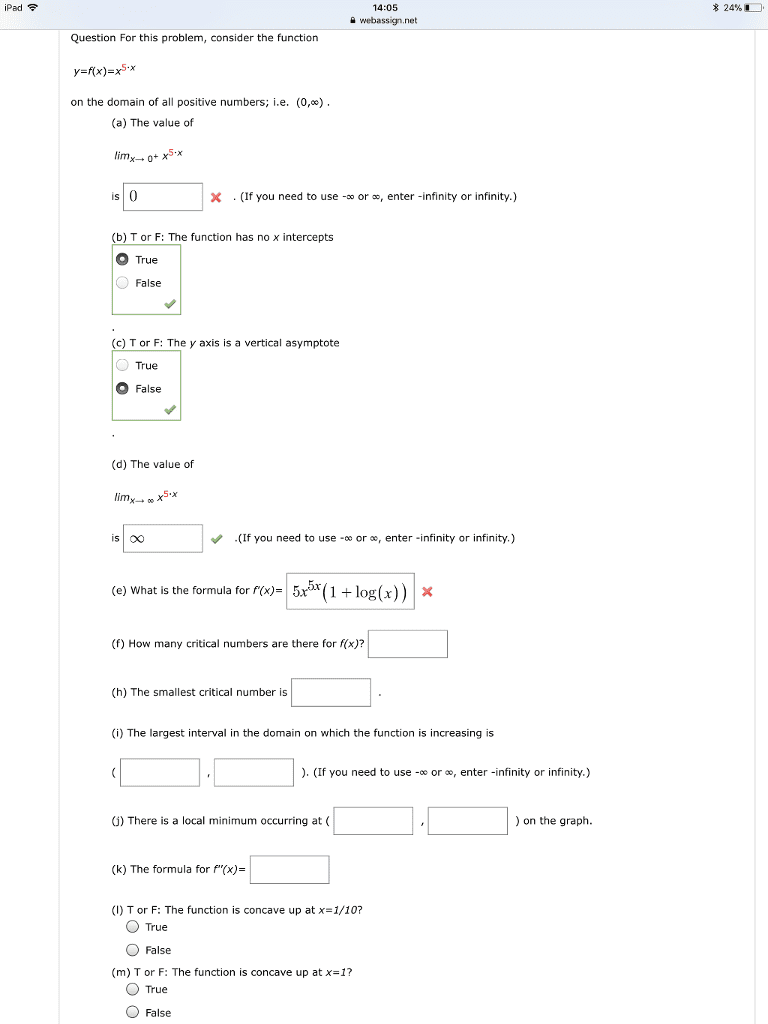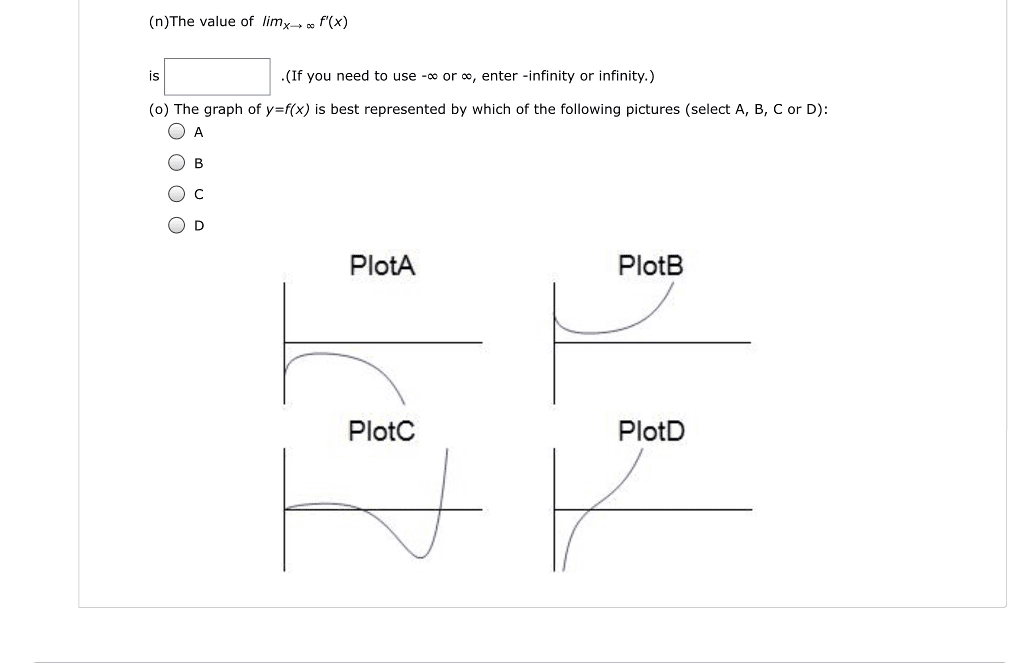1
0
watching
38
views13 Nov 2019

#iPadä»¤ 14:05 * 24% webassign.net Question For this problem, consider the function on the domain of all positive numbers; i.e. (0,Â«) (a) The value of is X . (If you need to use -oo or , enter -infinity or infinity.) (b) T or F: The function has no x intercepts True False (c) T or F: The y axis is a vertical asymptote True False (d) The value of "(If you need to use-oo orâ, enter-infinity or infinity.) (e) what is the formula for r(x),5x5x0+log(x)) Ã (f) How many critical numbers are there for f(x)? (h) The smallest critical number is (i) The largest interval in the domain on which the function is increasing is . (If you need to use - or , enter -infinity or infinity.) (i) There is a local minimum occurring at on the graph. (k) The formula for f"(x)= (I) T or F: The function is concave up at x=1/10? True False (m) T or F: The function is concave up at x=1? True False